# How to show a span element for each rows clicked in AngularJS?

A span element is used to group in-line elements in a document. It can be used to make a hook to a particular part of the document which may be subject to a particular action based on DOM events.
The span element can be used to highlight, show, hide or do any particular action on it based on a function.
Angular provides several directives by which the span element can be easily manipulated. Some of the examples are given below:

Approach 1: This is a basic rating giving HTML code. Here the span elements are the checked and checked star symbols.
The ng-show and ng-hide is used to show or hide the checked or unchecked star symbol. Here the click is used to manipulate the value of a variable which in turn shows the checked star symbol.

Syntax:

```<button ng-click="[A FUNCTION CALL] > Click! < button>
<span ng-show="[An boolean Expression] > The element < /span>```

Example:

 ` ` `<``html``> ` ` `  `<``head``> ` `    ``<``title``>Angular Span element on click ` `    ``<``script` `src``= ` `"https://ajax.googleapis.com/ajax/libs/angularjs/1.3.14/angular.min.js"``> ` `    `` ` `    ``<``link` `rel``=``"stylesheet"` `href``= ` `"https://cdnjs.cloudflare.com/ajax/libs/font-awesome/4.7.0/css/font-awesome.min.css"``> ` `    ``<``style``> ` `        ``.checked { ` `            ``color: orange; ` `        ``} ` `         `  `        ``.rateButton { ` `            ``border-radius: 12px; ` `            ``background-color: goldenrod; ` `        ``} ` `    `` ` ` ` ` `  `<``body``> ` `    ``<``div` `ng-app``=``"mainApp"` `         ``ng-controller``=``"RatingController"``> ` `        ``<``table``> ` `            ``<``tr``> ` `                ``<``td``>Rate Product ` `                ``` ` `  `                ``<``td``> ` `                    ``<``span` `ng-show="rating>=1" ` `                          ``ng-hide="rating<``1``"  ` `                          ``class``=``"fa fa-star checked"``> ` `                  `` ` `                    ``<``span` `ng-hide="rating>=1"  ` `                          ``ng-show="rating<``1``" ` `                          ``class``=``"fa fa-star unchecked"``> ` `                  `` ` ` `  `                    ``<``span` `ng-show="rating>=2"  ` `                          ``ng-hide="rating<``2``"  ` `                          ``class``=``"fa fa-star checked"``> ` `                  `` ` `                    ``<``span` `ng-hide="rating>=2" ` `                          ``ng-show="rating<``2``"  ` `                          ``class``=``"fa fa-star unchecked"``> ` `                  `` ` ` `  `                    ``<``span` `ng-show="rating>=3" ` `                          ``ng-hide="rating<``3``"  ` `                          ``class``=``"fa fa-star checked"``> ` `                  `` ` `                    ``<``span` `ng-hide="rating>=3"  ` `                          ``ng-show="rating<``3``"  ` `                          ``class``=``"fa fa-star unchecked"``> ` `                  `` ` ` `  `                    ``<``span` `ng-show="rating>=4"  ` `                          ``ng-hide="rating<``4``"  ` `                          ``class``=``"fa fa-star checked"``> ` `                  `` ` `                    ``<``span` `ng-hide="rating>=4" ` `                          ``ng-show="rating<``4``" ` `                          ``class``=``"fa fa-star unchecked"``> ` `                  `` ` ` `  `                    ``<``span` `ng-show="rating>=5"  ` `                          ``ng-hide="rating<``5``"  ` `                          ``class``=``"fa fa-star checked"``> ` `                  `` ` `                    ``<``span` `ng-hide="rating>=5" ` `                          ``ng-show="rating<``5``"  ` `                          ``class``=``"fa fa-star unchecked"``> ` `                  `` ` ` `  `                                `` ` ` `  `                                ``<``td``>  ` `                                    ``<``button` `ng-click``=``"Rating()"`  `                                            ``class``=``'rateButton'``> ` `                                      ``Click ` `                                  `` ` `                                `` ` `                            `` ` `                        `` ` `            `` ` ` `  `        ``<``script``> ` `            ``var app= angular.module("mainApp", []); ` `            ``app.controller('RatingController', function(\$scope){ ` `                ``\$scope.rating=0; ` `                ``\$scope.Rating=function(){ ` `                    ``\$scope.rating++;  ` `                ``}; ` `            ``}); ` `        `` ` `    `` ` ` `

Output:
Before click: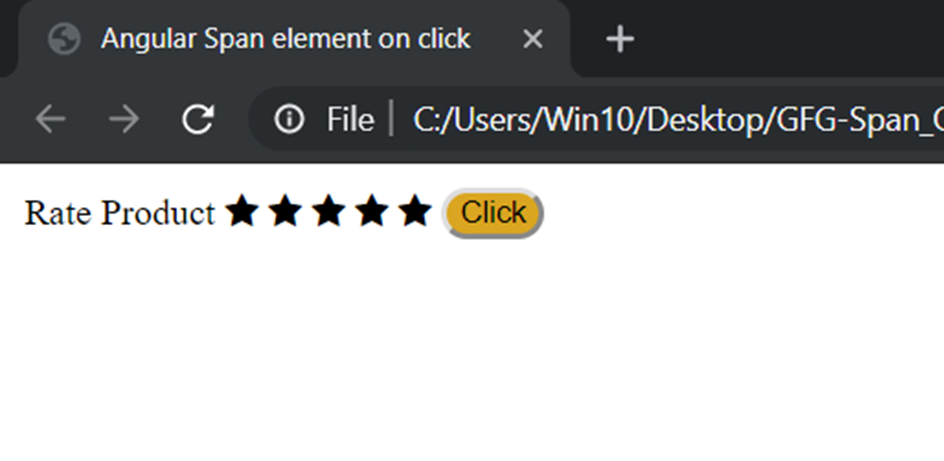After 3 clicks: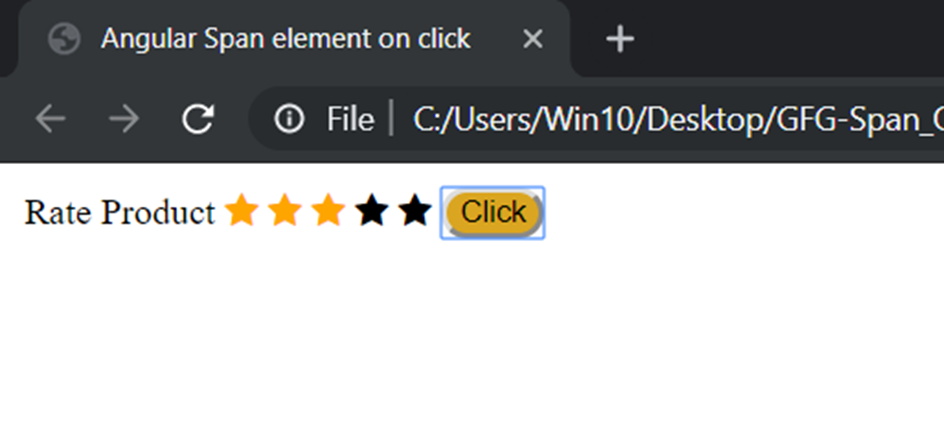Approach 2: This example shows how a part of the text can be hidden using the span and ng-if for selective viewers(here who know the password is 12345). Here the click event is done using event binding technique of angular. The Type Of event binding used is called Target event binding. The NgForm is used to trigger the functions using the event binding technique. In this technique, the event is bound in parenthesis () and the name of the event is the type of the button that is intended to create it.

Syntax:

```< form (nameOfEventBinder)="Function Call" > < /form >
< button type="nameOfEventBinder" > Click! < button >
< span ng-if="[An boolean Expression] > The element < /span >```

Example: test.html file:

 `<``table``> ` `    ``<``tr``> ` `        ``<``td``> The Hidden text is: ` `            ``<``span` `*``ngIf``=``"show==0"`  `                  ``class``=``"hidden"``>  ` `              ``text is hidden here ` `                `` ` `            ``<``span` `*``ngIf``=``"show==1"`  `                  ``class``=``"show"``>  ` `              ``This is the hidden text!! ` `                        `` ` `            ``<``span` `*``ngIf``=``"show==2"` `                  ``class``=``"error"``> ` `              ``Wrong Password ` `           `` ` `        `` ` `    `` ` `    ``<``tr``> ` `        ``<``td``> ` `            ``<``label` `for``=``"psw"``> Enter password to reveal text ` `            `` ` `            ``<``form` `(submit)="check(form)" ` `                  ``(reset)="reset(form)" ` `                  ``#``form``=``'ngForm'``> ` `                ``<``input` `type``=``"password"` `                       ``ngModel #``psw``=``"ngModel"`  `                       ``name``=``"psw"`  `                       ``placeholder``=``"Enter Password"`  `                       ``width``=``"20000"``> ` `                ``<``button` `type``=``"submit"``> submit ` `                `` ` `                ``<``button` `type``=``"reset"``> reset ` `                `` ` `            `` ` `        `` ` `    `` ` ` `

test.css file:

 `.``hidden``{ ` `    ``font-weight``: ``bold``; ` `} ` `.``show``{ ` `    ``color``: ``green``; ` `} ` `.error{ ` `    ``color``: ``red``; ` `} `

test.ts file:

 `import { Component } from ``'@angular/core'``; ` `import { NgForm } from ``'@angular/forms'``; ` `@Component({ ` `    ``selector: ``'app-test-html-component'``, ` `    ``templateUrl: ``'./test.html'``, ` `    ``styleUrls:[``'./test.css'``] ` `}) ` `export class TestComponent { ` ` `  `    ``show: any = 0; ` `    ``check(form: NgForm) { ` `        ``if` `(form.value.psw === ``'12345'``) { ` `            ``this``.show = 1; ` `        ``} ``else` `{ ` `            ``this``.show = 2; ` `        ``} ` `    ``} ` `    ``reset(form: NgForm) { ` `        ``form.value.psw = ``''``; ` `        ``this``.show = 0; ` `    ``} ` `} `

Output:
Initially: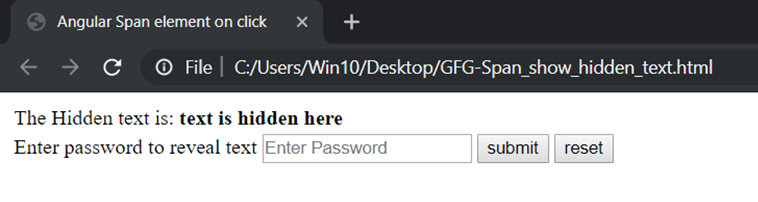After giving the correct password i.e. 12345: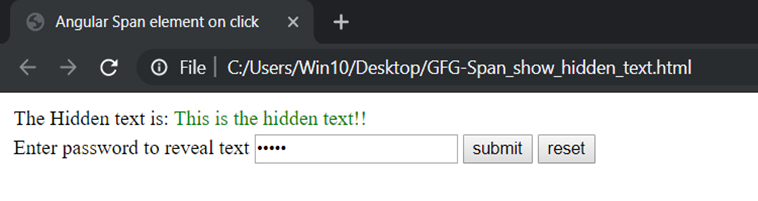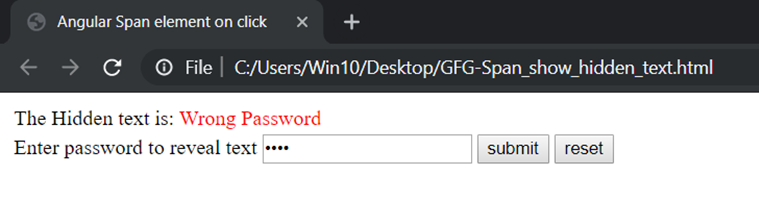My Personal Notes arrow_drop_upCheck out this Author's contributed articles.

If you like GeeksforGeeks and would like to contribute, you can also write an article using contribute.geeksforgeeks.org or mail your article to contribute@geeksforgeeks.org. See your article appearing on the GeeksforGeeks main page and help other Geeks.

Please Improve this article if you find anything incorrect by clicking on the "Improve Article" button below.